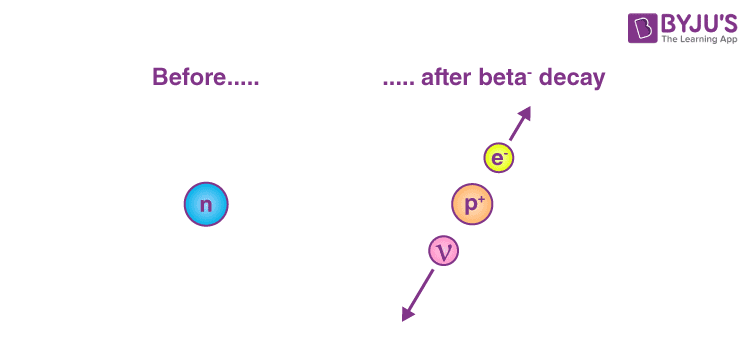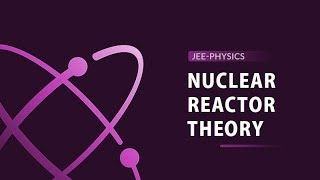Jet Set Go! All about Aeroplanes Jet Set Go! All about Aeroplanes

# Neutrino

Neutrinos are perplexing subatomic particles that could bear the key to answering many questions about our universe. In this article, let us familiarise ourselves with neutrinos and their properties.

## What is a Neutrino?

Neutrino is a subatomic particle with no electrical charge and a very small mass. They are difficult to detect because they have minimal interaction with matter. To detect neutrinos, very large and sensitive detectors are required.

Neutrino is a fermion because it is an elementary particle with a spin of ½ that interacts only via gravity and weak forces. The symbol ν denotes them.

Neutrinos are produced by different radioactive decays, such as

• beta decay of atomic nuclei
• when accelerated particle beams knock atoms
• artificial nuclear reactions in nuclear reactors or particle acceleratorsA neutrino, therefore, can be defined as a neutral fundamental particle with a mass close to zero and half-integral spin, that rarely reacts with the matter.

### Where do Neutrinos come from?

• The radioactive decay of primordial elements within the earth acts as a natural source of neutrinos; the decay generates an enormous flux of neutrinos.
• Roughly 2 per cent of the sun’s energy is carried away by neutrinos produced in fusion reactions.
• Supernovae are primarily a neutrino phenomenon because neutrinos are the only particles that can penetrate the very dense material produced in a collapsing star; only a small fraction of the available energy is converted to light.
• A considerable fraction of the universe’s dark matter may consist of primordial, Big Bang neutrinos.

## Neutrino Discovery

Wolfgang Pauli first hypothesised the existence of the neutrino in 1930. At that time, a problem emerged in beta decay when both energy and angular momentum were not conserved. But, Pauli suggested that if a non-interacting, neutral particle (a neutrino) were emitted, one could recover the conservation laws. However, the first detection of neutrinos did not happen until 1955, when Frederick Reines and Clyde Cowan documented anti-neutrinos emitted by a nuclear reactor.

## Types of NeutrinosNeutrinos are leptons because they have only been observed to interact through the weak force. These weak interactions produce neutrinos in three leptonic flavours:

• electron neutrino (νe)
• muon neutrino (vμ)
• tau neutrino (vτ

Each is associated with the corresponding charged leptons, the electron, the muon and tau, respectively.

## Properties of Neutrinos

The properties of neutrinos can be summarized as follows:

• Neutrinos belong to the family of leptons, and this family interacts through the weak force.
• There are three types or leptonic flavours of Neutrino: electron neutrino, muon neutrino and tau neutrino.
• A neutrino likewise has an antimatter component that is known as an antineutrino.
• The electromagnetic forces do not affect Neutrinos and hence, do not induce the ionization of matter.
• Neutrinos interact with matter only through weak interactive forces.
• A neutrino can pass through a massive number of atoms without provoking any reaction, and hence these are the most penetrating subatomic particles.
• The Neutrinos can also change one nucleus into another, and this process is used in a radiochemical neutrino detector.

## Neutrino Detector

A neutrino detector is a physics apparatus that is designed to study neutrinos. Neutrino sensors are usually constructed underground to sequester the detector from background radiation and cosmic rays.

Super-Kamiokande is a neutrino observatory built under Mount Ikeno in Japan. Super Kamiokande is a large volume of water surrounded by phototubes that observe for the Cherenkov radiation ejected when an incoming neutrino creates an electron or muon in the water. The Sudbury Neutrino Observatory is similar but uses heavy water as the detecting medium.

## Frequently Asked Questions – FAQs

### What is a Neutrino?

A neutrino is a neutral fundamental particle with a mass close to zero and a half-integral spin, that rarely reacts with the matter.

### Where is a Neutrino found?

​​Neutrinos can be found everywhere. They permeate the space all around us. They can be located throughout our galaxy.

### Where are Neutrino observatories placed?

Neutrino observatories are usually constructed underground to isolate the detector from background radiation and cosmic rays.

### How are Neutrinos formed?

Neutrinos are produced by different radioactive decays such as beta decay of atomic nuclei.

### What is an antineutrino?

The antimatter component of neutrino is known as an antineutrino.

Understand the parts and working of a nuclear reactor by watching the video!Stay tuned to BYJU’S and Fall in Love with Learning!